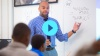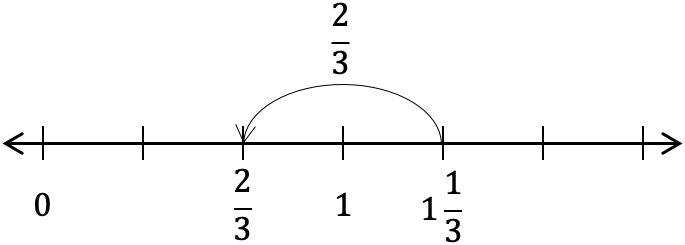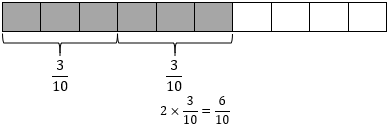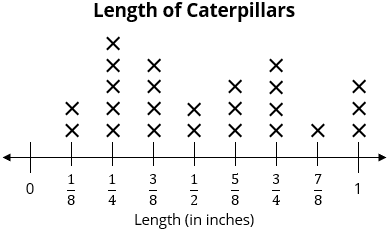# Fraction Operations

Students start to operate on fractions, learning how to add fractions with like denominators and multiply a whole number by any fraction.

Math

Unit 5

## Unit Summary

In this unit, 4th grade students begin their work with operating with fractions by understanding them as a sum of unit fractions or a product of a whole number and a unit fraction. Students will then add and subtract fractions with like denominators and multiply a whole number by a fraction, including mixed numbers. Students will apply this knowledge to word problems and line plots.

In 3rd Grade Math, students developed their understanding of the meaning of fractions, especially using the number line to make sense of fractions as numbers themselves. They also did some rudimentary work with equivalent fractions and comparison of fractions. In Unit 4, 4th grade students deepened this understanding of equivalence and comparison, learning the fundamental property that “multiplying the numerator and denominator of a fraction by the same non-zero whole number results in a fraction that represents the same number as the original fraction” (NF Progression, p. 6).

Thus, in this unit, armed with a deep understanding of fractions and their value, students start to operate on them for the first time. The unit is structured so that students build their understanding of fraction operations gradually, first working with the simplest case where the total is a fraction less than 1, then the case where the total is a fraction between 1 and 2 (to understand regrouping when operating in simple cases), and finally the case where the total is a fraction greater than 2. With each of these numerical cases, they first develop an understanding of non-unit fractions as sums and multiples of unit fractions. Next, they learn to add and subtract fractions. And finally, they apply these understandings to complex cases, such as word problems or fraction addition involving fractions where one denominator is a multiple of the other, which helps prepare students for similar work with decimal fractions in Unit 6. After working with all three numerical cases in the context of fraction addition and subtraction, they work with fraction multiplication, learning methods for multiplying a whole number by a fraction and a mixed number and using those skills in the context of word problems. Finally, students apply this unit’s work to the context of line plots. Students will solve problems by using information presented in line plots, requiring them to use their recently acquired skills of fraction addition, subtraction, and even multiplication, creating a contextual way for this supporting cluster content to support the major work of the grade. The unit provides lots of opportunity for students to reason abstractly and quantitatively (MP.2) and construct viable arguments and critique the reasoning of others (MP.3).

Students’ understanding of fractions is developed further in Unit 6, in which students explore decimal numbers via their relationship to decimal fractions, expressing a given quantity in both fraction and decimal forms (4.NF.5—7). Then, in 5th grade, students extend their understanding and ability with operations with fractions (5.NF.1—7), working with all cases of fraction addition, subtraction, and multiplication and the simple cases of division of a unit fraction by a whole number or vice versa. Students then develop a comprehensive understanding of and ability to compute fraction division problems in all cases in 6th grade (6.NS.1). Beyond these next few units and years, it is easy to find the application of this learning in nearly any mathematical subject in middle school and high school, from ratios and proportions in the middle grades to functional understanding in algebra.

Pacing: 25 instructional days (21 lessons, 2 flex days, 1 assessment day)

Fishtank Plus for Math

Unlock features to optimize your prep time, plan engaging lessons, and monitor student progress.## Assessment

The following assessments accompany Unit 5.

### Pre-Unit

Have students complete the Pre-Unit Assessment and Pre-Unit Student Self-Assessment before starting the unit. Use the Pre-Unit Assessment Analysis Guide to identify gaps in foundational understanding and map out a plan for learning acceleration throughout the unit.

### Mid-Unit

Have students complete the Mid-Unit Assessment after lesson 11.

### Post-Unit

Use the resources below to assess student understanding of the unit content and action plan for future units.

Expanded Assessment Package

Use student data to drive your planning with an expanded suite of unit assessments to help gauge students’ facility with foundational skills and concepts, as well as their progress with unit content.

## Unit Prep

### Intellectual Prep

Unit Launch

Prepare to teach this unit by immersing yourself in the standards, big ideas, and connections to prior and future content. Unit Launches include a series of short videos, targeted readings, and opportunities for action planning.#### Intellectual Prep for All Units

• Read and annotate “Unit Summary” and “Essential Understandings” portion of the unit plan.
• Do all the Target Tasks and annotate them with the “Unit Summary” and “Essential Understandings” in mind.
• Take the Post-Unit Assessment.

#### Unit-Specific Intellectual Prep

 number line Example: Use a number line to solve $$1\frac{1}{3}-\frac{2}{3}$$.tape diagram Example: Use a tape diagram to solve $$2\times \frac{3}{10}$$.line plot### Essential Understandings

• “The meaning of addition is the same for both fractions and whole numbers, even though algorithms for calculating their sums can be different. Just as the sum of $$4$$ and $$7$$ can be seen as the length of the segment obtained by joining together two segments of lengths 4 and 7, so the sum of $${{{{2\over3}}}}$$ and $${{{{8\over5}}}}$$ can be seen as the length of the segment obtained joining together two segments of length $${{{{2\over3}}}}$$ and $${{{{8\over5}}}}$$” (Progressions for the Common Core State Standards in Mathematics, 3-5 Numbers and Operations - Fractions, p. 7).
• Quantities cannot be added or subtracted if they do not have like units. Just like one cannot add 4 pencils and 3 bananas to have 7 of anything of meaning (unless one changes the unit of both to “objects”), the same applies for the units of fractions (their denominators). This explains why one must find a common denominator to be able to add fractions with unlike denominators when adding and subtracting fractions. Further, when you add or subtract quantities with like units, their units do not change. Just like one adds 5 bananas and 2 bananas and gets 7 bananas, one adds 5 eighths and 2 eighths and gets 7 eighths.
• “Converting a mixed number to a fraction should not be viewed as a separate technique to be learned by rote, but simply as a case of fraction addition. Similarly, converting an improper fraction to a mixed number is a matter of decomposing the fraction into a sum of a whole number and a number less than 1” (Progressions for the Common Core State Standards in Mathematics, 3-5 Numbers and Operations - Fractions, p. 8).
• “It is possible to over-emphasize the importance of simplifying fractions. There is no mathematical reason why fractions must be written in simplified form, although it may be convenient to do so in some cases” (Progressions for the Common Core State Standards in Mathematics, 3-5 Numbers and Operations - Fractions, p. 6). Thus, students should not be expected to simplify fractions in all cases where it’s possible to do so.

### Vocabulary

fraction greater than one

mixed number

To see all the vocabulary for Unit 5, view our 4th Grade Vocabulary Glossary.

### Materials

• Buttons (About 20 per small group) — These should be of various diameters. There are other options to use in place of this material. See Lesson 20 Anchor Task 3 for more information.
• Rulers (1 per student) — These should measure to the nearest eighth inch and ideally the 0 inch mark is not flush with the end of the ruler.

## Unit Practice

Word Problems and Fluency Activities

Access daily word problem practice and our content-aligned fluency activities created to help students strengthen their application and fluency skills.## Lesson Map

Topic A: Building, Adding, and Subtracting Fractions Less Than or Equal to 1

Topic B: Building, Adding, and Subtracting Fractions Less Than 2

Topic C: Building, Adding, and Subtracting Fractions Greater Than or Equal to 2

Topic D: Multiplication of Fractions

Topic E: Line Plots

## Common Core Standards

Key

Major Cluster

Supporting Cluster

### Core Standards

#### Measurement and Data

• 4.MD.B.4 — Make a line plot to display a data set of measurements in fractions of a unit (1/2, 1/4, 1/8). Solve problems involving addition and subtraction of fractions by using information presented in line plots. For example, from a line plot find and interpret the difference in length between the longest and shortest specimens in an insect collection.

#### Number and Operations—Fractions

• 4.NF.B.3 — Understand a fraction a/b with a > 1 as a sum of fractions 1/b.
• 4.NF.B.3.A — Understand addition and subtraction of fractions as joining and separating parts referring to the same whole.
• 4.NF.B.3.B — Decompose a fraction into a sum of fractions with the same denominator in more than one way, recording each decomposition by an equation. Justify decompositions, e.g., by using a visual fraction model. Examples: 3/8 = 1/8 + 1/8 + 1/8 ; 3/8 = 1/8 + 2/8 ; 2 1/8 = 1 + 1 + 1/8 = 8/8 + 8/8 + 1/8.
• 4.NF.B.3.C — Add and subtract mixed numbers with like denominators, e.g., by replacing each mixed number with an equivalent fraction, and/or by using properties of operations and the relationship between addition and subtraction.
• 4.NF.B.3.D — Solve word problems involving addition and subtraction of fractions referring to the same whole and having like denominators, e.g., by using visual fraction models and equations to represent the problem.
• 4.NF.B.4 — Apply and extend previous understandings of multiplication to multiply a fraction by a whole number.
• 4.NF.B.4.A — Understand a fraction a/b as a multiple of 1/b. For example, use a visual fraction model to represent 5/4 as the product 5 × (1/4), recording the conclusion by the equation 5/4 = 5 × (1/4).
• 4.NF.B.4.B — Understand a multiple of a/b as a multiple of 1/b, and use this understanding to multiply a fraction by a whole number. For example, use a visual fraction model to express 3 × (2/5) as 6 × (1/5), recognizing this product as 6/5. (In general, n × (a/b) = (n × a)/b.)
• 4.NF.B.4.C — Solve word problems involving multiplication of a fraction by a whole number, e.g., by using visual fraction models and equations to represent the problem. For example, if each person at a party will eat 3/8 of a pound of roast beef, and there will be 5 people at the party, how many pounds of roast beef will be needed? Between what two whole numbers does your answer lie?

• 3.MD.B.4

• 3.NF.A.1
• 3.NF.A.2
• 4.NF.A.1
• 4.NF.A.2

• 4.MD.A.2
• 5.MD.B.2

• 4.NF.C.5
• 5.NF.A.1
• 5.NF.B.4
• 5.NF.B.7

### Standards for Mathematical Practice

• CCSS.MATH.PRACTICE.MP1 — Make sense of problems and persevere in solving them.

• CCSS.MATH.PRACTICE.MP2 — Reason abstractly and quantitatively.

• CCSS.MATH.PRACTICE.MP3 — Construct viable arguments and critique the reasoning of others.

• CCSS.MATH.PRACTICE.MP4 — Model with mathematics.

• CCSS.MATH.PRACTICE.MP5 — Use appropriate tools strategically.

• CCSS.MATH.PRACTICE.MP6 — Attend to precision.

• CCSS.MATH.PRACTICE.MP7 — Look for and make use of structure.

• CCSS.MATH.PRACTICE.MP8 — Look for and express regularity in repeated reasoning.

Unit 4

Fraction Equivalence and Ordering

Unit 6

Decimal Fractions

## Request a Demo

See all of the features of Fishtank in action and begin the conversation about adoption.

Yes

No

### Any other information you would like to provide about your school?